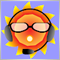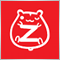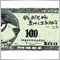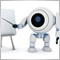# 請問非常多層的for迴圈如何加速呢？36

for(int a=0;a<=10;a++){

for(int b=0;b<=10;b++){

for(int c=0;c<=10;c++){

for(int d=0;d<=10;d++){

for(int e=0;e<=10;e++){

for(int f=0;f<=10;f++){

...........

}

}

}

}

}

}3091628591971